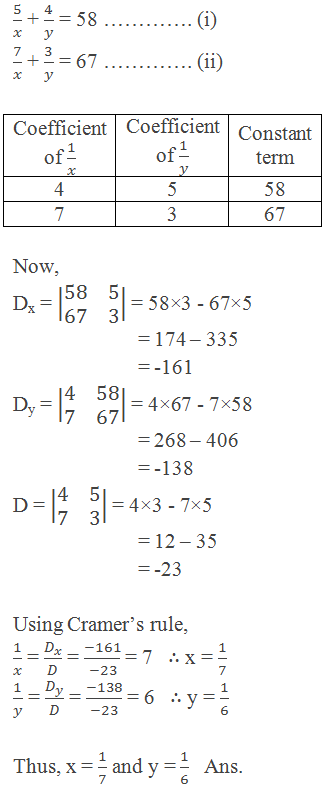# Cramer’s RuleGabriel Cramer (1704-1752) was a great mathematician from Geneva. He showed his promise in mathematics from an early age. At the age of 18, he received his doctorate and at the age of 20, he was the Co-chair of mathematics at the University of Geneva.

English inventor Charles Babbage (1792-1871) knew a method for solving linear systems called Cramer’s Rule, in honor of the Swiss geometer Gabriel Cramer (1704-1752). Cramer’s rule was simple but involved numerous multiplications for large systems. Babbage designed a machine, called the “difference engine”, that consisted of toothed wheels on shafts for performing these multiplications. Despite the fact that only one-seventh of the functions ever worked, Babbage’s invention demonstrated how complex calculations could be handled mechanically. In 1944, scientists at IBM used the lessons of the difference engine to create the world’s first computer.

********************

10 Math Problems officially announces the release of Quick Math Solver, an Android App on the Google Play Store for students around the world.

********************## Solving System of Linear Equations in Two Variables Using Determinants

Determinants can be used to solve a linear system of equations in two variables. In general, such a system appears as

a1x + b1y = c1 …………… (i)

a2x + b2y = c2 …………… (ii)

Let’s first solve this system by the elimination method. We can solve for x by eliminating y from the equations. Multiply the first equation by b2 and the second equation by –b1, then add the two equations: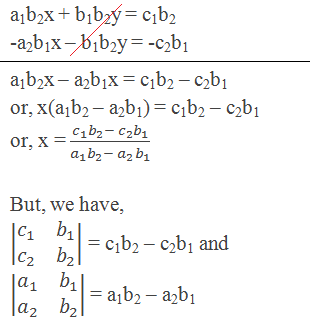So, we can express the value of x as the quotient of two determinants.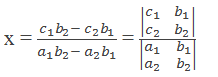Similarly, multiply equation (i) by a2 and equation (ii) by –a1, then add the equations: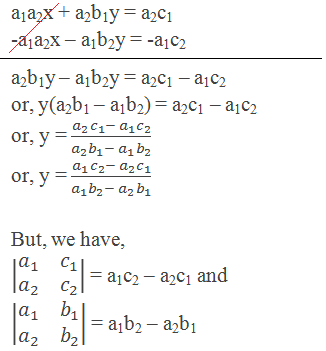So, we can express the value of y as the quotient of two determinants.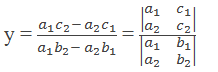This method of using determinants to solve the linear system is called Cramer’s Rule.

## Cramer’s Rule in Summary

If a1x + b1y = c1 and a2x + b2y = c2 are system of linear equations, then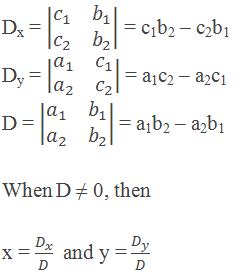This method of solving a system of linear equations is called Cramer’s Rule.

## Worked Out Examples

Example 1: Solve simultaneous equations 5x – 4y = 2 and 6x – 5y = 1 by using Cramer’s rule.

Solution: Here,

5x – 4y = 2 …………. (i)

6x – 5y = 1 …………. (ii)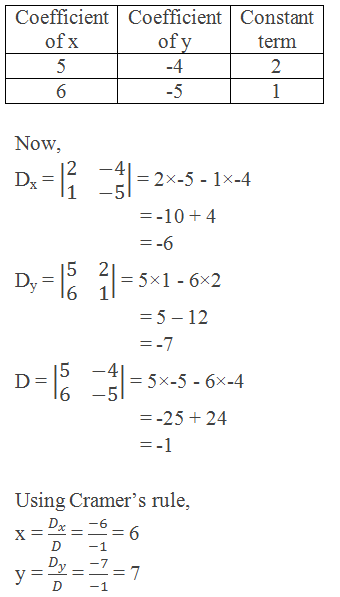Thus, x = 6 and y = 7   Ans.

Example 2: Use Cramer’s rule to solve the equations: x + 2y = 7 and 2x – y = 4.

Solution: Here,

x + 2y = 7 …………. (i)

2x – y = 4 …………. (ii)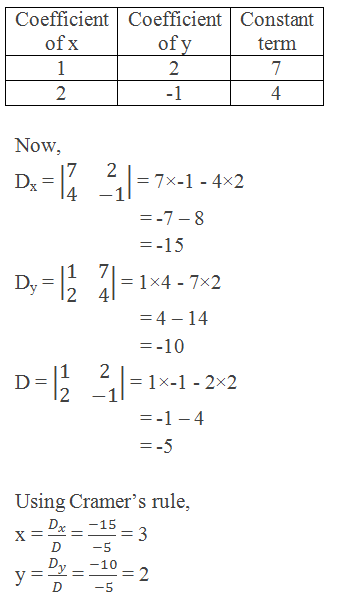Thus, x = 3 and y = 2   Ans.

Example 3: Solve the following system of equations using Cramer’s rule: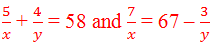Solution: Here,